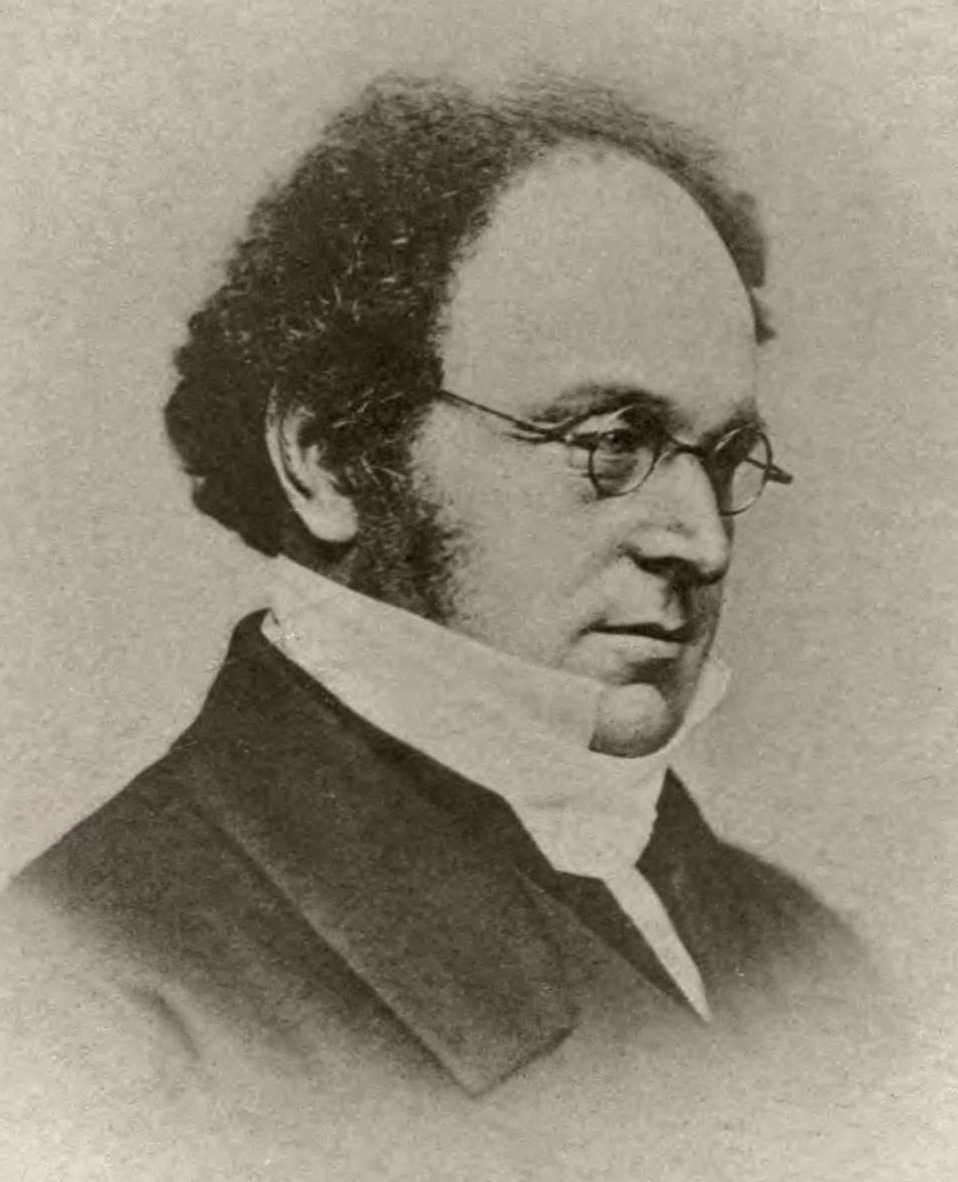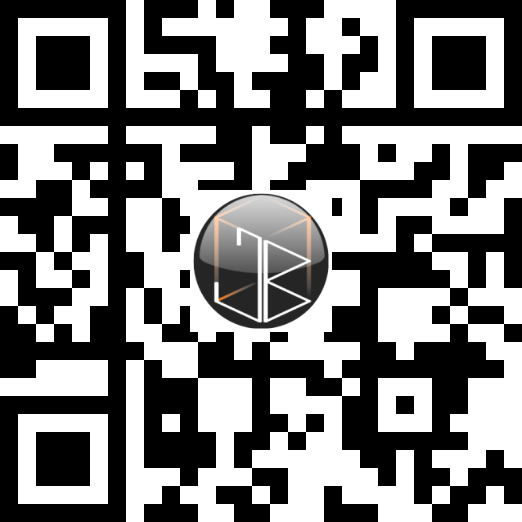# Part 3.3Conditions

A major part of any program is checking conditions. This allows programs to decide what sequence to follow.

Condition checking is used to evaluate which branch of code the program should run, or at least where the computer should look for the next piece of code.

## What is a condition?

A condition is something that needs to be met or satisfied to be able to evaluate to true. When a condition is satisfied the result of the condition is a Boolean true.

For example:

A teacher tells his pupils that all pupils are allowed to leave the classroom on the condition that all of the chairs are pushed under and all of the computers are logged off.

The condition here is that all chairs are pushed under and that all of the computers are logged off. The result of this is satisfied when both of these conditions, collectively the condition, have been met.

With programming, the concept remains the same - a condition must be met before the program decides which branch of code to follow.

For example:

YASS
$condition = true and false  The previous condition will obviously evaluate to false. A simple condition is one that is built up using the simple operators shown below: Mathematical operator Operator Name Example = = or == Equal to 5 = 5 > > Greater than 5 > 4 < < Less than 5 < 6 >= >= Greater than or equal to 5 >= 5 <= <= Less than or equal to 5 <= 5 ¬ != Not equal to 5 != 6 A complex condition involves using logical conjunction or disjunction, that is and (∧) and or (∨) and is built-up of multiple simple conditions. And and or, or logical conjunction and disjunction use simple rules that if read like English would make sense. These rules are composed using truth tables. Truth tables are normally composed of two values, A and B, as well as some statements. In this case the truth table shows what the result of both the A & B and the A | B statements. In all cases, A and B can themselves be either a simple value (that is a true or false) or simple (or complex) statements themselves. A B A ∧ B A ∨ B T T T T T F F T F T F T F F F F ## If statements Computers use conditions often to make decisions about which branch of code to follow. An if statement is the construct that does this in most languages. An if statement is also called selection since it selects a path or syntax tree to follow based on a decision. Within an if statement, there is a condition that is evaluated and either one or multiple routes that the code can follow: YASS if($condition == true)
print("Followed if statement")
else
print("Went a different route")
end if


If statements also often have else if statements within them. These statements offer another check before going down the else route.

YASS
$a = 13 if($a >= 20)
print("Greater than 20")
else if(\$a >= 10))
print("Greater than 10")
else
print("Less than 10")
end if


## De Morgan's Law

In the 19th century, George Boole theorised and proved many theories related to logic and eventually created Boolean logic.Augustus De Morgan

A British mathematician named Augustus De Morgan expanded on Boole's theories and came up with a now well used law. Computer science obviously had grassroots at this point, but much of it was based on theories and laws like this.

De Morgan's Law is one of the fundamentals to the basics of computer science. The law refers to the transformation that conjunction and disjunction in logic can go through when a statement is negated. It involves switching all operators - both logical (AND, OR, NOT) and comparators (>, <, >=, <=, ==, !=).

The following table shows what operators become what:

Initial operator 'Flipped' operator
AND OR
OR AND
NOT NOT NOT
> <=
< >=
>= <
<= >=
== !=
!= ==

For example:

¬(P ∧ Q) == ¬P ∨ ¬Q

Putting this into our truth tables proves De Moran's Law works and testing both of these statements also proves this:

¬(P ∧ Q)
P = True
Q = True
¬P = False
¬Q = False

P ∧ Q = True
¬(P ∧ Q) == False
¬P ∨ ¬Q
P = True
Q = True
¬P = False
¬Q = False

¬P ∨ ¬Q = False

Because of this, it is clear that ANDs become ORs when they are negated and ORs become ANDs. This can then be applied to conditions in programming to prove that the negation of an AND condition will become the equivalent to flipping both the operands and changing the AND operator to an OR:

(n > 0) AND (n < 30)
n = 15
(n > 0) = True
(n < 30) = True
True AND True == True

¬(n <= 0) OR ¬(n >= 30)
n = 15
(n <= 0) = False
(n >= 30) = False

¬(n <= 0) = True
¬(n >= 30) = True
True OR True == TrueCode preview
Feedback 👍
Comments are sent via email to me.